# Sampling

Currently, there are 8 functions associated with the sample verb in the sgsR package:

Algorithm Description Reference
sample_srs() Simple random
sample_systematic() Systematic
sample_strat() Stratified Queinnec, White, & Coops (2021)
sample_nc() Nearest centroid Melville & Stone (2016)
sample_clhs() Conditioned Latin hypercube Minasny & McBratney (2006)
sample_balanced() Balanced sampling Grafström, A. Lisic, J (2018)
sample_ahels() Adapted hypercube evaluation of a legacy sample Malone, Minasny, & Brungard (2019)
sample_existing() Sub-sampling an existing sample

## sample_srs

We have demonstrated a simple example of using the sample_srs() function in vignette("sgsR"). We will demonstrate additional examples below.

raster

The input required for sample_srs() is a raster. This means that sraster and mraster are supported for this function.

#--- perform simple random sampling ---#
sample_srs(raster = sraster, # input sraster
nSamp = 200, # number of desired sample units
plot = TRUE) # plot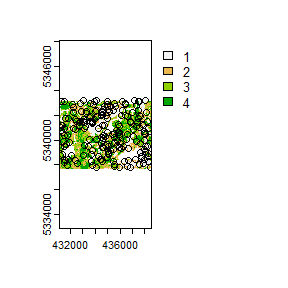#> Simple feature collection with 200 features and 0 fields
#> Geometry type: POINT
#> Dimension:     XY
#> Bounding box:  xmin: 431150 ymin: 5337770 xmax: 438490 ymax: 5343230
#> CRS:           +proj=utm +zone=17 +ellps=GRS80 +units=m +no_defs
#> First 10 features:
#>                  geometry
#> 1  POINT (432270 5342210)
#> 2  POINT (434970 5341730)
#> 3  POINT (434410 5338430)
#> 4  POINT (438030 5338850)
#> 5  POINT (438290 5338930)
#> 6  POINT (431670 5339670)
#> 7  POINT (435910 5340630)
#> 8  POINT (431210 5341030)
#> 9  POINT (431490 5340350)
#> 10 POINT (434670 5342490)
sample_srs(raster = mraster, # input mraster
nSamp = 200, # number of desired sample units
access = access, # define access road network
mindist = 200, # minimum distance sample units must be apart from one another
buff_inner = 50, # inner buffer - no sample units within this distance from road
buff_outer = 200, # outer buffer - no sample units further than this distance from road
plot = TRUE) # plot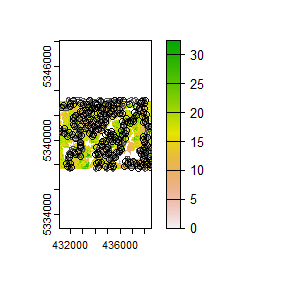#> Simple feature collection with 200 features and 0 fields
#> Geometry type: POINT
#> Dimension:     XY
#> Bounding box:  xmin: 431110 ymin: 5337750 xmax: 438530 ymax: 5343230
#> CRS:           +proj=utm +zone=17 +ellps=GRS80 +units=m +no_defs
#> First 10 features:
#>                  geometry
#> 1  POINT (434890 5342470)
#> 2  POINT (433070 5342030)
#> 3  POINT (437530 5337750)
#> 4  POINT (435750 5338990)
#> 5  POINT (433850 5340490)
#> 6  POINT (434810 5341430)
#> 7  POINT (436810 5338170)
#> 8  POINT (433790 5342570)
#> 9  POINT (435430 5342550)
#> 10 POINT (435330 5342290)

## sample_systematic

The sample_systematic() function applies systematic sampling across an area with the cellsize parameter defining the resolution of the tessellation. The tessellation shape can be modified using the square parameter. Assigning TRUE (default) to the square parameter results in a regular grid and assigning FALSE results in a hexagonal grid.

The location of sample units can also be adjusted using the locations parameter, where centers takes the center, corners takes all corners, and random takes a random location within each tessellation. Random start points and translations are applied when the function is called.

#--- perform grid sampling ---#
sample_systematic(raster = sraster, # input sraster
cellsize = 1000, # grid distance
plot = TRUE) # plot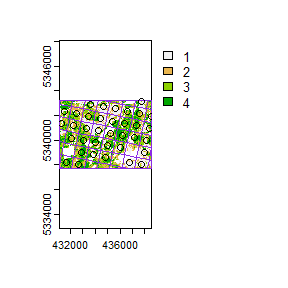#> Simple feature collection with 36 features and 0 fields
#> Geometry type: POINT
#> Dimension:     XY
#> Bounding box:  xmin: 431290 ymin: 5338021 xmax: 438546.1 ymax: 5343122
#> CRS:           +proj=utm +zone=17 +ellps=GRS80 +units=m +no_defs
#> First 10 features:
#>                    geometry
#> 1  POINT (431683.6 5338217)
#> 2  POINT (432664.3 5338021)
#> 3    POINT (432860 5339002)
#> 4  POINT (433840.6 5338806)
#> 5  POINT (434821.3 5338611)
#> 6  POINT (436782.6 5338219)
#> 7  POINT (437763.3 5338023)
#> 8    POINT (432075 5340178)
#> 9  POINT (433055.7 5339983)
#> 10 POINT (434036.3 5339787)
#--- perform grid sampling ---#
sample_systematic(raster = sraster, # input sraster
cellsize = 500, # grid distance
square = FALSE, # hexagonal tessellation
location = "random", # randomly sample within tessellation
plot = TRUE) # plot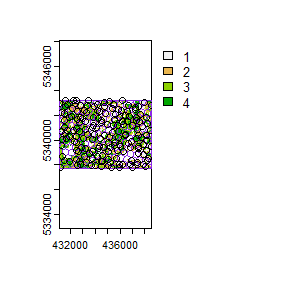#> Simple feature collection with 167 features and 0 fields
#> Geometry type: POINT
#> Dimension:     XY
#> Bounding box:  xmin: 431105.6 ymin: 5337762 xmax: 438524.2 ymax: 5343239
#> CRS:           +proj=utm +zone=17 +ellps=GRS80 +units=m +no_defs
#> First 10 features:
#>                    geometry
#> 1  POINT (431603.5 5343188)
#> 2  POINT (431105.6 5343004)
#> 3  POINT (432200.2 5343239)
#> 4  POINT (431755.4 5342863)
#> 5  POINT (432461.8 5343237)
#> 6  POINT (431602.4 5342708)
#> 7  POINT (432387.9 5342949)
#> 8  POINT (431291.8 5342275)
#> 9  POINT (432111.6 5342545)
#> 10 POINT (432792.9 5342813)
sample_systematic(raster = sraster, # input sraster
cellsize = 500, # grid distance
access = access, # define access road network
buff_outer = 200, # outer buffer - no sample units further than this distance from road
square = FALSE, # hexagonal tessellation
location = "corners", # take corners instead of centers
plot = TRUE)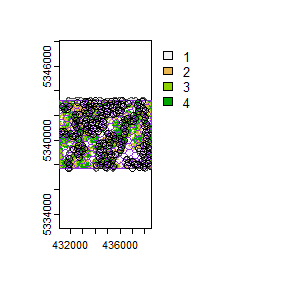#> Simple feature collection with 629 features and 0 fields
#> Geometry type: POINT
#> Dimension:     XY
#> Bounding box:  xmin: 431114.2 ymin: 5337735 xmax: 438470.7 ymax: 5343228
#> CRS:           +proj=utm +zone=17 +ellps=GRS80 +units=m +no_defs
#> First 10 features:
#>                    geometry
#> 1  POINT (438470.7 5337887)
#> 2  POINT (438470.7 5337887)
#> 3  POINT (438302.6 5338121)
#> 4  POINT (438421.8 5338384)
#> 5  POINT (438421.8 5338384)
#> 6  POINT (438253.7 5338619)
#> 7  POINT (438372.9 5338882)
#> 8  POINT (438302.6 5338121)
#> 9  POINT (438470.7 5337887)
#> 10 POINT (437896.1 5337830)

## sample_strat

The sample_strat() contains two methods to perform sampling:

• "Queinnec" - Hierarchical sampling using a focal window to isolate contiguous groups of stratum pixels, which was originally developed by Martin Queinnec.

• "random" - Traditional stratified random sampling. This method ignores much of the functionality of the algorithm to allow users the capability to use standard stratified random sampling approaches without the use of a focal window to locate contiguous stratum cells.

### method = "Queinnec"

Queinnec, M., White, J. C., & Coops, N. C. (2021). Comparing airborne and spaceborne photon-counting LiDAR canopy structural estimates across different boreal forest types. Remote Sensing of Environment, 262(August 2020), 112510.

This algorithm uses moving window (wrow and wcol parameters) to filter the input sraster to prioritize sample unit allocation to where stratum pixels are spatially grouped, rather than dispersed individuals across the landscape.

Sampling is performed using 2 rules:

• Rule 1 - Sample within spatially grouped stratum pixels. Moving window defined by wrow and wcol.

• Rule 2 - If no additional sample units exist to satisfy desired sample size(nSamp), individual stratum pixels are sampled.

The rule applied to a select each sample unit is defined in the rule attribute of output samples. We give a few examples below:

#--- perform stratified sampling random sampling ---#
sample_strat(sraster = sraster, # input sraster
nSamp = 200, # desired sample size
plot = TRUE) # plot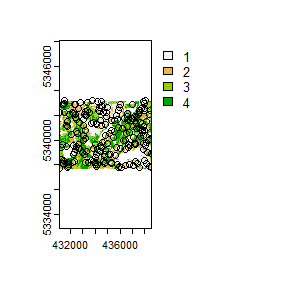#> Simple feature collection with 200 features and 3 fields
#> Geometry type: POINT
#> Dimension:     XY
#> Bounding box:  xmin: 431110 ymin: 5337730 xmax: 438550 ymax: 5343210
#> CRS:           NA
#> First 10 features:
#>    strata type  rule               geometry
#> x       1  new rule1 POINT (437910 5339750)
#> x1      1  new rule2 POINT (434350 5342330)
#> x2      1  new rule2 POINT (437890 5341070)
#> x3      1  new rule2 POINT (435010 5339750)
#> x4      1  new rule2 POINT (436130 5337790)
#> x5      1  new rule2 POINT (434170 5339230)
#> x6      1  new rule2 POINT (437870 5343110)
#> x7      1  new rule2 POINT (438470 5340630)
#> x8      1  new rule2 POINT (437950 5342430)
#> x9      1  new rule2 POINT (434130 5341330)

In some cases, users might want to include an existing sample within the algorithm. In order to adjust the total number of sample units needed per stratum to reflect those already present in existing, we can use the intermediate function extract_strata().

This function uses the sraster and existing sample units and extracts the stratum for each. These sample units can be included within sample_strat(), which adjusts total sample units required per class based on representation in existing.

#--- extract strata values to existing samples ---#
e.sr <- extract_strata(sraster = sraster, # input sraster
existing = existing) # existing samples to add strata value to

TIP!

sample_strat() requires the sraster input to have an attribute named strata and will give an error if it doesn’t.

sample_strat(sraster = sraster, # input sraster
nSamp = 200, # desired sample size
access = access, # define access road network
existing = e.sr, # existing sample with strata values
mindist = 200, # minimum distance sample units must be apart from one another
buff_inner = 50, # inner buffer - no sample units within this distance from road
buff_outer = 200, # outer buffer - no sample units further than this distance from road
plot = TRUE) # plot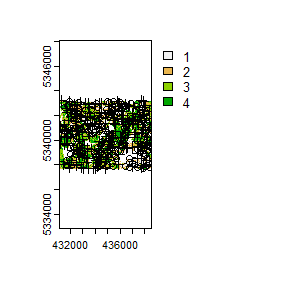#> Simple feature collection with 400 features and 3 fields
#> Geometry type: POINT
#> Dimension:     XY
#> Bounding box:  xmin: 431130 ymin: 5337730 xmax: 438550 ymax: 5343230
#> CRS:           NA
#> First 10 features:
#>    strata     type     rule               geometry
#> 1       1 existing existing POINT (434350 5342270)
#> 2       1 existing existing POINT (434330 5340910)
#> 3       1 existing existing POINT (433470 5342990)
#> 4       1 existing existing POINT (434810 5337750)
#> 5       1 existing existing POINT (435870 5342990)
#> 6       1 existing existing POINT (434290 5339190)
#> 7       1 existing existing POINT (437910 5343150)
#> 8       1 existing existing POINT (435170 5342410)
#> 9       1 existing existing POINT (433910 5341250)
#> 10      1 existing existing POINT (435910 5338870)

The code in the example above defined the mindist parameter, which specifies the minimum euclidean distance that new sample units must be apart from one another.

Notice that the sample units have type and rule attributes which outline whether they are existing or new, and whether rule1 or rule2 were used to select them. If type is existing (a user provided existing sample), rule will be existing as well as seen above.

sample_strat(sraster = sraster, # input
nSamp = 200, # desired sample size
access = access, # define access road network
existing = e.sr, # existing samples with strata values
include = TRUE, # include existing sample in nSamp total
buff_outer = 200, # outer buffer - no samples further than this distance from road
plot = TRUE) # plot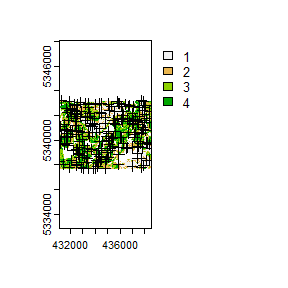#> Simple feature collection with 200 features and 3 fields
#> Geometry type: POINT
#> Dimension:     XY
#> Bounding box:  xmin: 431130 ymin: 5337730 xmax: 438550 ymax: 5343230
#> CRS:           NA
#> First 10 features:
#>    strata     type     rule               geometry
#> 1       1 existing existing POINT (434350 5342270)
#> 2       1 existing existing POINT (434330 5340910)
#> 3       1 existing existing POINT (433470 5342990)
#> 4       1 existing existing POINT (434810 5337750)
#> 5       1 existing existing POINT (435870 5342990)
#> 6       1 existing existing POINT (434290 5339190)
#> 7       1 existing existing POINT (437910 5343150)
#> 8       1 existing existing POINT (435170 5342410)
#> 9       1 existing existing POINT (433910 5341250)
#> 10      1 existing existing POINT (435910 5338870)

The include parameter determines whether existing sample units should be included in the total sample size defined by nSamp. By default, the include parameter is set as FALSE.

### method = "random

Stratified random sampling with equal probability for all cells (using default algorithm values for mindist and no use of access functionality). In essence this method perform the sample_srs algorithm for each stratum separately to meet the specified sample size.

#--- perform stratified sampling random sampling ---#
sample_strat(sraster = sraster, # input sraster
method = "random", #stratified random sampling
nSamp = 200, # desired sample size
plot = TRUE) # plot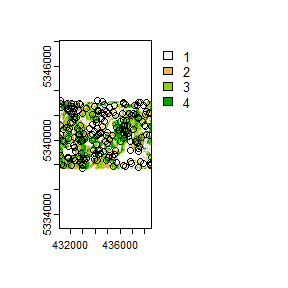#> Simple feature collection with 200 features and 1 field
#> Geometry type: POINT
#> Dimension:     XY
#> Bounding box:  xmin: 431110 ymin: 5337710 xmax: 438550 ymax: 5343210
#> Projected CRS: UTM Zone 17, Northern Hemisphere
#> First 10 features:
#>    strata               geometry
#> 1       1 POINT (435130 5338750)
#> 2       1 POINT (432290 5340230)
#> 3       1 POINT (434170 5340930)
#> 4       1 POINT (436290 5337950)
#> 5       1 POINT (435010 5339750)
#> 6       1 POINT (436850 5339190)
#> 7       1 POINT (436270 5343010)
#> 8       1 POINT (436930 5338130)
#> 9       1 POINT (431790 5342530)
#> 10      1 POINT (434750 5342090)

## sample_nc

sample_nc() function implements the Nearest Centroid sampling algorithm described in Melville & Stone (2016). The algorithm uses kmeans clustering where the number of clusters (centroids) is equal to the desired sample size (nSamp).

Cluster centers are located, which then prompts the nearest neighbour mraster pixel for each cluster to be selected (assuming default k parameter). These nearest neighbours are the output sample units.

#--- perform simple random sampling ---#
sample_nc(mraster = mraster, # input
nSamp = 25, # desired sample size
plot = TRUE)
#> K-means being performed on 3 layers with 25 centers.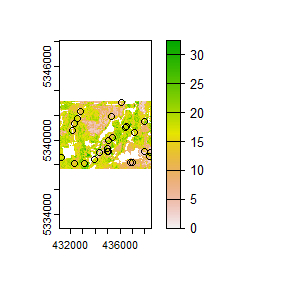#> Simple feature collection with 25 features and 4 fields
#> Geometry type: POINT
#> Dimension:     XY
#> Bounding box:  xmin: 431290 ymin: 5338090 xmax: 438430 ymax: 5343070
#> CRS:           +proj=utm +zone=17 +ellps=GRS80 +units=m +no_defs
#> First 10 features:
#>        zq90 pzabove2  zsd kcenter               geometry
#> 79070 12.10     50.8 3.36       1 POINT (438430 5339010)
#> 35122 11.90     89.6 2.73       2 POINT (432290 5341350)
#> 48419 17.80     80.3 4.91       3 POINT (437130 5340650)
#> 85035 16.20     84.7 4.14       4 POINT (438370 5338690)
#> 95922 14.60     90.7 3.44       5 POINT (432310 5338090)
#> 73674 23.20     88.9 6.68       6 POINT (434950 5339290)
#> 94291  8.38     85.8 1.83       7 POINT (436990 5338190)
#> 41299 19.10     61.9 5.70       8 POINT (436470 5341030)
#> 77930  6.88     14.2 1.77       9 POINT (438010 5339070)
#> 3237  13.60     74.2 3.44      10 POINT (436150 5343070)

Altering the k parameter leads to a multiplicative increase in output sample units where total output samples = $$nSamp * k$$.

#--- perform simple random sampling ---#
samples <- sample_nc(mraster = mraster, # input
k = 2, # number of nearest neighbours to take for each kmeans center
nSamp = 25, # desired sample size
plot = TRUE)
#> K-means being performed on 3 layers with 25 centers.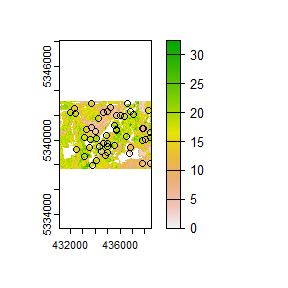#--- total samples = nSamp * k (25 * 2) = 50 ---#
nrow(samples)
#>  50

Visualizing what the kmeans centers and sample units looks like is possible when using details = TRUE. The $kplot output provides a quick visualization of where the centers are based on a scatter plot of the first 2 layers in mraster. Notice that the centers are well distributed in covariate space and chosen sample units are the closest pixels to each center (nearest neighbours). #--- perform simple random sampling with details ---# details <- sample_nc(mraster = mraster, # input nSamp = 25, # desired sample number details = TRUE) #> K-means being performed on 3 layers with 25 centers. #--- plot ggplot output ---# details$kplot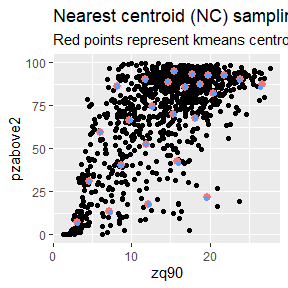## sample_clhs

sample_clhs() function implements conditioned Latin hypercube (clhs) sampling methodology from the clhs package.

TIP!

A number of other functions in the sgsR package help to provide guidance on clhs sampling including calculate_pop() and calculate_lhsOpt(). Check out these functions to better understand how sample numbers could be optimized.

The syntax for this function is similar to others shown above, although parameters like iter, which define the number of iterations within the Metropolis-Hastings process are important to consider. In these examples we use a low iter value for efficiency. Default values for iter within the clhs package are 10,000.

sample_clhs(mraster = mraster, # input
nSamp = 200, # desired sample size
plot = TRUE, # plot
iter = 100) # number of iterations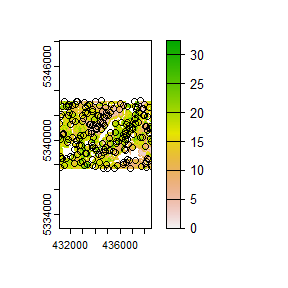The cost parameter defines the mraster covariate, which is used to constrain the clhs sampling. An example could be the distance a pixel is from road access (e.g. from calculate_distance() see example below), terrain slope, the output from calculate_coobs(), or many others.

#--- cost constrained examples ---#
#--- calculate distance to access layer for each pixel in mr ---#
mr.c <- calculate_distance(raster = mraster, # input
access = access, # define access road network
plot = TRUE) # plot
#>
|---------|---------|---------|---------|
=========================================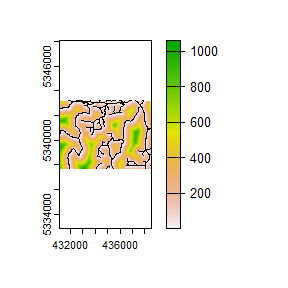sample_clhs(mraster = mr.c, # input
nSamp = 250, # desired sample size
iter = 100, # number of iterations
cost = "dist2access", # cost parameter - name defined in calculate_distance()
plot = TRUE) # plot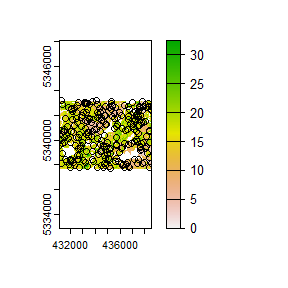## sample_balanced

The sample_balanced() algorithm performs a balanced sampling methodology from the stratifyR / SamplingBigData packages.

sample_balanced(mraster = mraster, # input
nSamp = 200, # desired sample size
plot = TRUE) # plot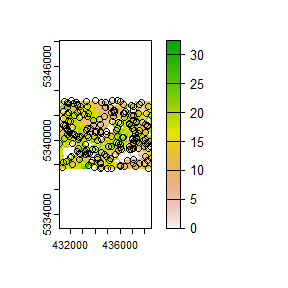#> Simple feature collection with 200 features and 0 fields
#> Geometry type: POINT
#> Dimension:     XY
#> Bounding box:  xmin: 431110 ymin: 5337730 xmax: 438550 ymax: 5343230
#> CRS:           +proj=utm +zone=17 +ellps=GRS80 +units=m +no_defs
#> First 10 features:
#>                  geometry
#> 1  POINT (435830 5343230)
#> 2  POINT (432110 5343210)
#> 3  POINT (434010 5343190)
#> 4  POINT (435370 5343190)
#> 5  POINT (436050 5343170)
#> 6  POINT (433270 5343150)
#> 7  POINT (431470 5343130)
#> 8  POINT (434810 5343090)
#> 9  POINT (437730 5343090)
#> 10 POINT (431770 5343070)
sample_balanced(mraster = mraster, # input
nSamp = 100, # desired sample size
algorithm = "lcube", # algorithm type
access = access, # define access road network
buff_inner = 50, # inner buffer - no sample units within this distance from road
buff_outer = 200) # outer buffer - no sample units further than this distance from road
#> Simple feature collection with 100 features and 0 fields
#> Geometry type: POINT
#> Dimension:     XY
#> Bounding box:  xmin: 431610 ymin: 5337730 xmax: 438550 ymax: 5343150
#> CRS:           +proj=utm +zone=17 +ellps=GRS80 +units=m +no_defs
#> First 10 features:
#>                  geometry
#> 1  POINT (437890 5338130)
#> 2  POINT (432750 5339490)
#> 3  POINT (438150 5339590)
#> 4  POINT (434610 5342250)
#> 5  POINT (435130 5337730)
#> 6  POINT (434570 5339210)
#> 7  POINT (433990 5342910)
#> 8  POINT (434350 5341950)
#> 9  POINT (433730 5342730)
#> 10 POINT (437950 5338930)

## sample_ahels

The sample_ahels() function performs the adapted Hypercube Evaluation of a Legacy Sample (ahels) algorithm usingexisting sample data and an mraster. New sample units are allocated based on quantile ratios between the existing sample and mraster covariate dataset.

This algorithm was adapted from that presented in the paper below, which we highly recommend.

Malone BP, Minansy B, Brungard C. 2019. Some methods to improve the utility of conditioned Latin hypercube sampling. PeerJ 7:e6451 DOI 10.7717/peerj.6451

This algorithm:

1. Determines the quantile distributions of existing sample units and mraster covariates.

2. Determines quantiles where there is a disparity between sample units and covariates.

3. Prioritizes sampling within those quantile to improve representation.

To use this function, user must first specify the number of quantiles (nQuant) followed by either the nSamp (total number of desired sample units to be added) or the threshold (sampling ratio vs. covariate coverage ratio for quantiles - default is 0.9) parameters.

#--- remove type variable from existing  - causes plotting issues ---#

existing <- existing %>% select(-type)

sample_ahels(mraster = mraster,
existing = existing, # existing sample
plot = TRUE) # plot
#> Simple feature collection with 241 features and 7 fields
#> Geometry type: POINT
#> Dimension:     XY
#> Bounding box:  xmin: 431130 ymin: 5337730 xmax: 438550 ymax: 5343230
#> CRS:           +proj=utm +zone=17 +ellps=GRS80 +units=m +no_defs
#> First 10 features:
#>      type.x  zq90 pzabove2  zsd strata type.y  rule               geometry
#> 1  existing 10.20     45.5 2.74      1    new rule1 POINT (434350 5342270)
#> 2  existing  4.24     56.3 0.87      1    new rule2 POINT (434330 5340910)
#> 3  existing 10.60     30.5 2.94      1    new rule2 POINT (433470 5342990)
#> 4  existing 10.50     92.5 2.49      1    new rule2 POINT (434810 5337750)
#> 5  existing 10.10     34.4 2.55      1    new rule2 POINT (435870 5342990)
#> 6  existing  1.94      0.1 0.20      1    new rule2 POINT (434290 5339190)
#> 7  existing  3.98      5.7 0.96      1    new rule2 POINT (437910 5343150)
#> 8  existing 10.50     43.9 2.81      1    new rule2 POINT (435170 5342410)
#> 9  existing  3.23     20.0 0.73      1    new rule2 POINT (433910 5341250)
#> 10 existing  6.93     25.5 1.85      1    new rule2 POINT (435910 5338870)

TIP!

Notice that no threshold, nSamp, or nQuant were defined. That is because the default setting for threshold = 0.9 and nQuant = 10.

The first matrix output shows the quantile ratios between the sample and the covariates. A value of 1.0 indicates that the sample is representative of quantile coverage. Values > 1.0 indicate over representation of sample units, while < 1.0 indicate under representation.

sample_ahels(mraster = mraster,
existing = existing, # existing sample
nQuant = 20, # define 20 quantiles
nSamp = 300) # desired sample size
#> Simple feature collection with 500 features and 7 fields
#> Geometry type: POINT
#> Dimension:     XY
#> Bounding box:  xmin: 431130 ymin: 5337730 xmax: 438550 ymax: 5343230
#> CRS:           +proj=utm +zone=17 +ellps=GRS80 +units=m +no_defs
#> First 10 features:
#>      type.x  zq90 pzabove2  zsd strata type.y  rule               geometry
#> 1  existing 10.20     45.5 2.74      1    new rule1 POINT (434350 5342270)
#> 2  existing  4.24     56.3 0.87      1    new rule2 POINT (434330 5340910)
#> 3  existing 10.60     30.5 2.94      1    new rule2 POINT (433470 5342990)
#> 4  existing 10.50     92.5 2.49      1    new rule2 POINT (434810 5337750)
#> 5  existing 10.10     34.4 2.55      1    new rule2 POINT (435870 5342990)
#> 6  existing  1.94      0.1 0.20      1    new rule2 POINT (434290 5339190)
#> 7  existing  3.98      5.7 0.96      1    new rule2 POINT (437910 5343150)
#> 8  existing 10.50     43.9 2.81      1    new rule2 POINT (435170 5342410)
#> 9  existing  3.23     20.0 0.73      1    new rule2 POINT (433910 5341250)
#> 10 existing  6.93     25.5 1.85      1    new rule2 POINT (435910 5338870)

Notice that the total number of samples is 500. This value is the sum of existing units (200) and number of sample units defined by nSamp = 300.

## sample_existing

Acknowledging that existing sample networks exist is important. There is significant investment into these samples, and in order to keep inventories up-to-date, we often need to collect new data at these locations. The sample_existing algorithm provides a method for sub-sampling an existing sample network should the financial / logistical resources not be available to collect data at all sample units. The algorithm leverages latin hypercube sampling using the clhs package to effectively sample within an existing network.

The algorithm has two fundamental approaches:

1. Sample exclusively using the sample network and the attributes it contains.

2. Should raster information be available and co-located with the sample, use these data as population values to improve sub-sampling of existing.

Much like the sample_clhs() algorithm, users can define a cost parameter, which will be used to constrain sub-sampling. A cost parameter is a user defined metric/attribute such as distance from roads (e.g. calculate_distance()), elevation, etc.

### Basic sub-sampling of existing

First we can create an existing sample for our example. Lets imagine we have a dataset of ~900 samples, and we know we only have resources to sample 300 of them. We have some ALS data available (mraster), which we will use as our distributions to sample within.

#--- generate existing samples and extract metrics ---#
existing <- sample_srs(raster = mraster, nSamp = 900, plot = TRUE)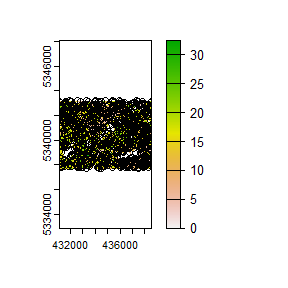Now lets sub-sample.

#--- sub sample using ---#
e <- existing %>%
extract_metrics(mraster = mraster, existing = .)

sample_existing(existing = e, nSamp = 300, plot = TRUE)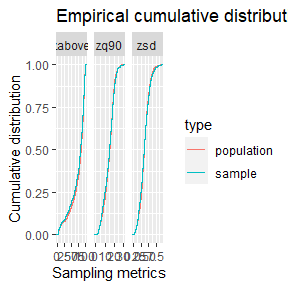#> Simple feature collection with 300 features and 3 fields
#> Geometry type: POINT
#> Dimension:     XY
#> Bounding box:  xmin: 431110 ymin: 5337770 xmax: 438550 ymax: 5343230
#> CRS:           +proj=utm +zone=17 +ellps=GRS80 +units=m +no_defs
#> First 10 features:
#>      zq90 pzabove2  zsd               geometry
#> 116  7.24     64.5 1.64 POINT (437050 5337810)
#> 66  12.10     98.5 2.77 POINT (437490 5337810)
#> 843 16.40     96.5 2.78 POINT (434550 5340150)
#> 413 10.50     67.9 2.89 POINT (437810 5341870)
#> 616 24.00     89.0 6.56 POINT (437330 5340390)
#> 172 20.00     84.8 6.41 POINT (435910 5341150)
#> 740 17.90     92.3 3.37 POINT (436410 5341510)
#> 211  2.95     12.3 0.50 POINT (438370 5338350)
#> 683 17.10     82.1 3.66 POINT (432070 5341930)
#> 451 23.30     99.4 6.02 POINT (437910 5340650)

TIP!

Notice that we used extract_metrics() after creating our existing. If the user provides a raster for the algorithm this isn’t neccesary (its done internally). If only sample units are given, attributes must be provided and sampling will be conducted on all included attributes.

We see from the output that we get 300 sample units that are a sub-sample of existing. The plotted output shows cumulative frequency distributions of the population (all existing samples) and the sub-sample (the 300 samples we requested).

### Sub-sampling using raster distributions

Our systematic sample of ~900 plots is fairly comprehensive, however we can generate a true population distribution through the inclusion of the ALS metrics in the sampling process. The metrics will be included in internal latin hypercube sampling to help guide sub-sampling of existing.

#--- sub sample using ---#
sample_existing(existing = existing, # our existing sample
nSamp = 300, # desired sample size
raster = mraster, # include mraster metrics to guide sampling of existing
plot = TRUE) # plot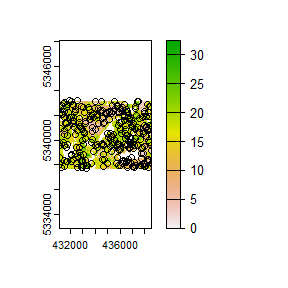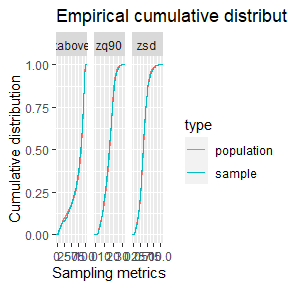#> Simple feature collection with 300 features and 3 fields
#> Geometry type: POINT
#> Dimension:     XY
#> Bounding box:  xmin: 431110 ymin: 5337730 xmax: 438550 ymax: 5343210
#> CRS:           +proj=utm +zone=17 +ellps=GRS80 +units=m +no_defs
#> First 10 features:
#>      zq90 pzabove2  zsd               geometry
#> 370 11.10     71.0 3.05 POINT (432470 5339430)
#> 674 12.30     77.3 3.10 POINT (437050 5339910)
#> 811 10.90     87.4 2.77 POINT (436230 5338110)
#> 112  8.34     64.7 1.95 POINT (437130 5338190)
#> 21  13.70     39.5 3.84 POINT (434990 5339250)
#> 509 17.80     95.6 3.65 POINT (432750 5339410)
#> 899 10.80     55.0 2.96 POINT (435850 5339230)
#> 245 14.60     84.4 3.45 POINT (437930 5341910)
#> 429  4.09     12.1 0.88 POINT (434270 5341330)
#> 211  2.95     12.3 0.50 POINT (438370 5338350)

The sample distribution again mimics the population distribution quite well! Now lets try using a cost variable to constrain the sub-sample.

#--- create distance from roads metric ---#
dist <- calculate_distance(raster = mraster, access = access)
#>
|---------|---------|---------|---------|
=========================================

#--- sub sample using ---#
sample_existing(existing = existing, # our existing sample
nSamp = 300, # desired sample size
raster = dist, # include mraster metrics to guide sampling of existing
cost = 4, # either provide the index (band number) or the name of the cost layer
plot = TRUE) # plot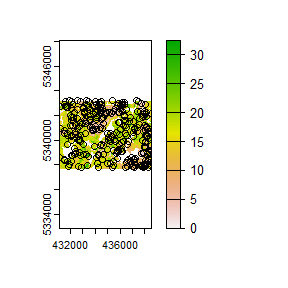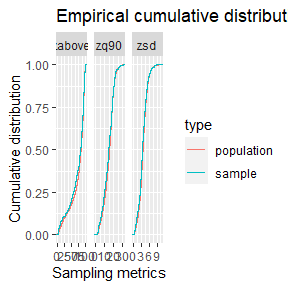#> Simple feature collection with 300 features and 4 fields
#> Geometry type: POINT
#> Dimension:     XY
#> Bounding box:  xmin: 431130 ymin: 5337770 xmax: 438550 ymax: 5343230
#> CRS:           +proj=utm +zone=17 +ellps=GRS80 +units=m +no_defs
#> First 10 features:
#>      zq90 pzabove2  zsd dist2access               geometry
#> 855  8.83     10.0 2.43   113.75852 POINT (438290 5340510)
#> 759  8.57     71.2 2.30    23.82931 POINT (435850 5342230)
#> 343 15.70     61.2 3.91   236.92489 POINT (432950 5341370)
#> 459  8.01     53.6 2.62   200.56034 POINT (434010 5343150)
#> 621 19.10     62.0 4.67   515.15800 POINT (437550 5341290)
#> 508 12.10     66.5 3.33    62.57196 POINT (433930 5342770)
#> 241 11.20     91.1 2.95    56.52950 POINT (432650 5339970)
#> 358 16.50     90.6 3.03   241.76470 POINT (436530 5340350)
#> 268 24.70     96.6 6.37    50.58752 POINT (438450 5338650)
#> 178 22.40     90.4 6.03   128.62834 POINT (438110 5341930)

Finally, should the user wish to further constrain the sample based on access like other sampling approaches in sgsR that is also possible.

#--- ensure access and existing are in the same CRS ---#

sf::st_crs(existing) <- sf::st_crs(access)

#--- sub sample using ---#
sample_existing(existing = existing, # our existing sample
nSamp = 300, # desired sample size
raster = dist, # include mraster metrics to guide sampling of existing
cost = 4, # either provide the index (band number) or the name of the cost layer
access = access, # roads layer
buff_inner = 50, # inner buffer - no sample units within this distance from road
buff_outer = 300, # outer buffer - no sample units further than this distance from road
plot = TRUE) # plot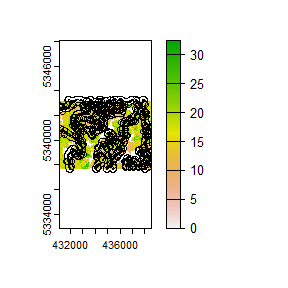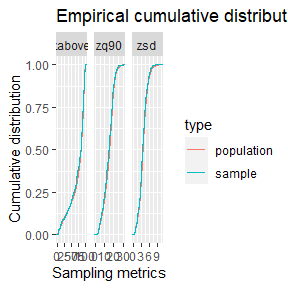#> Simple feature collection with 300 features and 4 fields
#> Geometry type: POINT
#> Dimension:     XY
#> Bounding box:  xmin: 431130 ymin: 5337770 xmax: 438550 ymax: 5343230
#> Projected CRS: UTM_Zone_17_Northern_Hemisphere
#> First 10 features:
#>     zq90 pzabove2  zsd dist2access               geometry
#> 105 18.6     82.7 4.55   124.72271 POINT (436450 5341170)
#> 102 17.2     86.6 4.08   137.29634 POINT (433330 5342630)
#> 410 20.1     92.5 4.21   162.21921 POINT (432330 5340990)
#> 132 18.7     91.7 4.48    52.74159 POINT (432790 5340390)
#> 75  17.8     26.3 5.68    99.88939 POINT (435210 5339870)
#> 170 15.7     61.2 3.91   236.92489 POINT (432950 5341370)
#> 204 22.1     92.9 6.53   164.78472 POINT (432990 5339690)
#> 229 14.9     89.8 3.55   195.25787 POINT (432190 5341190)
#> 337 17.8     70.5 4.38   225.34776 POINT (435770 5338570)
#> 194 22.0     82.2 6.29   153.59011 POINT (431770 5342870)

TIP!

The greater constraints we add to sampling, the less likely we will have strong correlations between the population and sample, so its always important to understand these limitations and plan accordingly.